{{ message }}

# marciok / Mu

It's a Swift playground explaining how to create a tiny programming language named Mu

## Files

Failed to load latest commit information.
Type
Name
Commit time

# Mu

It's a playground explaining how to create a tiny programming language (Mu).

You can download the playground here or check the source code live here

# Writing Your Own Programming Language

You don't need a CS degree to write a programing language, you just need to understand 3 basic steps.

## The Language: Mu(μ)

Mu is a minimal language, that is consisted by a postfix operator, a binary operation and one digit numbers.

### Examples:

`(s 2 4)` or `(s (s 4 5) 4)` or `(s (s 4 5) (s 3 2))`...

## The Steps: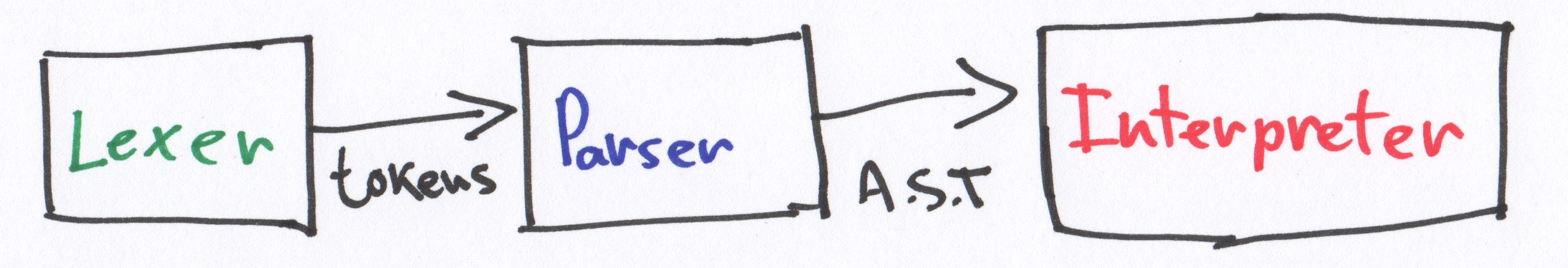# Lexer

"In computer science, lexical analysis is the process of converting a sequence of characters into a sequence of tokens (strings with an identified "meaning"). A program that performs lexical analysis may be called a lexer, tokenizer, or scanner (though "scanner" is also used to refer to the first stage of a lexer). Such a lexer is generally combined with a parser, which together analyze the syntax of programming languages..." -Wikipedia

The idea is to transform an array of charaters into an array of tokens (strings with an identified "meaning")

## Example: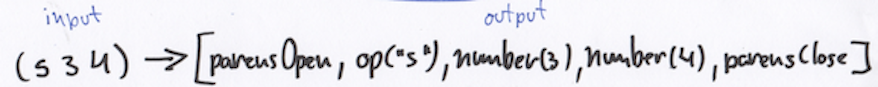Because `Mu` is so small--only one character operator and numbers--you can simply iterate over the input and check each character.

```enum Token {
case parensOpen
case op(String)
case number(Int)
case parensClose
}

struct Lexer {

static func tokenize(_ input: String) -> [Token] {
return input.characters.flatMap {
switch \$0 {
default:
if "0"..."9" ~= \$0 {
}
}

return nil
}
}
}

let input = "(s (s 4 5) 4)"
let tokens = Lexer.tokenize(input)
```

# Parser

Parsing or syntactic analysis is the process of analysing a string of symbols, either in natural language or in computer languages, conforming to the rules of a formal grammar... -Wikipedia

## Grammar:

`expression: parensOpen operator primaryExpression primaryExpression parensClose`

`primaryExpression: expression | number`

`parensOpen: "("`

`parensClose: ")"`

`operator: "s"`

`number: [0-9]`

`Mu`'s grammar is a context-free grammar, that means it describes all possible strings in the language. The parser will start from the top (root of the generated tree) and it will go until the lowest node.

Tip: the code should be a direct representation of the grammar

``````func parseExpression() -> ExpressionNode {
...
firstPrimaryExpression = parsePrimaryExpression()
secondPrimaryExpression = parsePrimaryExpression()
...
}

func parsePrimaryExpression() -> PrimaryExpressionNode {
return parseExpression() || parseNumber()
}
``````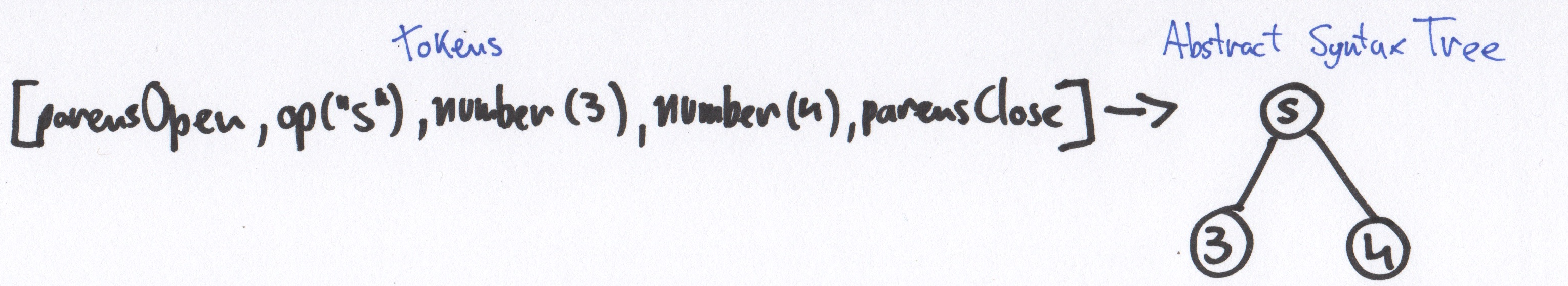```indirect enum PrimaryExpressionNode {
case number(Int)
case expression(ExpressionNode)
}

struct ExpressionNode {
var op: String
var firstExpression: PrimaryExpressionNode
var secondExpression: PrimaryExpressionNode
}

struct Parser {

var index = 0
let tokens: [Token]
init(tokens: [Token]) {
self.tokens = tokens
}

mutating func popToken() -> Token {
let token = tokens[index]
index += 1

}

mutating func peekToken() -> Token {
}

mutating func parse() throws -> ExpressionNode {
return try parseExpression()
}

mutating func parseExpression() throws -> ExpressionNode {
guard case .parensOpen = popToken() else {
throw ParsingError.unexpectedToken
}
guard case let Token.op(_operator) = popToken() else {
throw ParsingError.unexpectedToken
}

let firstExpression = try parsePrimaryExpression()
let secondExpression = try parsePrimaryExpression()

guard case .parensClose = popToken() else {
throw ParsingError.unexpectedToken
}

return ExpressionNode(op: _operator, firstExpression: firstExpression, secondExpression: secondExpression)
}

mutating func parsePrimaryExpression() throws -> PrimaryExpressionNode {
switch peekToken() {
case .number:
return try parseNumber()
case .parensOpen:
let expressionNode = try parseExpression()

return PrimaryExpressionNode.expression(expressionNode)
default:
throw ParsingError.unexpectedToken
}
}

mutating func parseNumber() throws -> PrimaryExpressionNode {
guard case let Token.number(n) = popToken() else { throw ParsingError.unexpectedToken }

return PrimaryExpressionNode.number(n)
}

}

//MARK: Utils

extension ExpressionNode: CustomStringConvertible {
public var description: String {
return "\(op) -> [\(firstExpression), \(secondExpression)]"
}
}
extension PrimaryExpressionNode: CustomStringConvertible {
public var description: String {
switch self {
case .number(let n): return n.description
case .expression(let exp): return exp.description
}
}
}

let input = "(s 2 (s 3 5))"
let tokens = Lexer.tokenize(input)
var parser = Parser(tokens: tokens)
var ast = try! parser.parse()
```

# Interpreter

"In computer science, an interpreter is a computer program that directly executes, i.e. performs, instructions written in a programming or scripting language, without previously compiling them into a machine language program." -Wikipedia

## Example:

`Mu`'s interpreter will walk through its A.S.T and compute a value by applying an operator to the children nodes.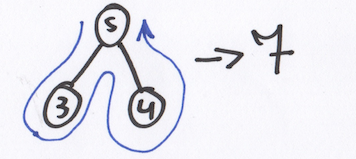```enum InterpreterError: Error {
case unknownOperator
}

struct Interpreter {
static func eval(_ expression: ExpressionNode) throws -> Int {
let firstEval = try eval(expression.first)
let secEval = try eval(expression.second)

if expression.op == "s" {
return firstEval + secEval
}

throw InterpreterError.unknownOperator
}

static func eval(_ prim: PrimaryExpressionNode) throws -> Int {
switch prim {
case .expression(let exp):
return try eval(exp)
case .number(let n):
return Int(n)
}
}

}

let input = "(s (s 5 2) 4)"
let tokens = Lexer.tokenize(input)
var parser = Parser(tokens: tokens)

let ast = try! parser.parse()
try! Interpreter.eval(ast)```

# Conclusion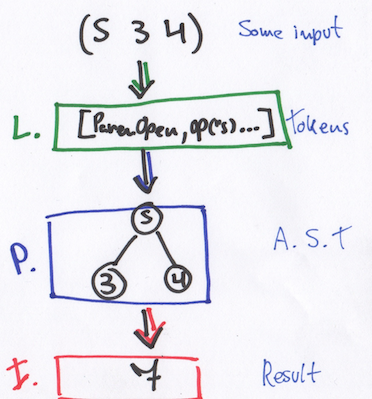• Given an input `let input = "(s (s 4 5) 4)`
• Extract an array of tokens (Lexing) `let tokens = Lexer.tokenize(input)`
• Parse the given tokens into a tree (Parsing)
``````var parser = Parser(tokens: tokens)
let ast = try! parser.parse()
``````
• And walk through this tree, and compute the values contained inside a node (Interpreting) `let result = try! Interpreter.eval(ast)`

### Resources

It's a Swift playground explaining how to create a tiny programming language named Mu

1.0.1 Latest
Nov 11, 2016

## Packages 0

No packages published

•
•

## Languages

You can’t perform that action at this time.